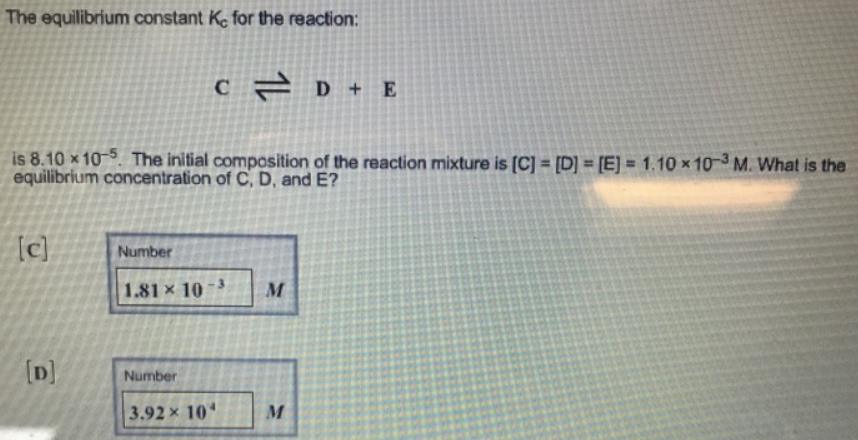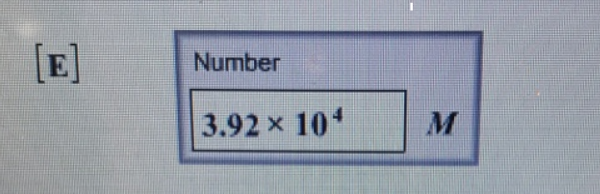# Problem: The equilibrium constant Kc for the reaction:C ⇌ D + E is 8.10 x10-5. The initial composition of the reaction mixture is [C} = [D] = [E] =   1.10x10^3 M. What is the equilibrium concentration of C, D, and E?

###### FREE Expert Solution
83% (83 ratings)###### Problem Details

The equilibrium constant Kc for the reaction:

C ⇌ D + E

is 8.10 x10-5. The initial composition of the reaction mixture is [C} = [D] = [E] =   1.10x10^3 M. What is the equilibrium concentration of C, D, and E?